#2017 iT 邦幫忙鐵人賽
DAY 24
1
Big Data

## Day24 R語言機器學習之羅吉斯迴歸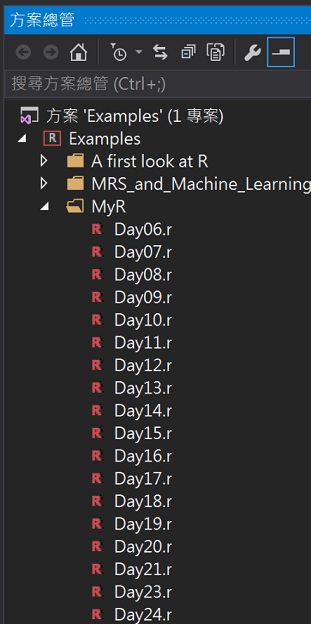## 資料集說明及簡單整理:

``````install.packages("AER")
library(AER)

#(1)載入creditcard資料集(包含1,319筆觀察測試，共有12個變數)
data(CreditCard)
#觀察資料欄位

#假設我們只要以下欄位(card:是否核准卡片、信用貶弱報告數、年齡、收入(美金)、自有住宅狀況、往來時間)
bankcard <- subset(CreditCard, select = c(card, reports, age, income, owner,months))

#將是否核准卡片轉換為0/1數值
bankcard\$card <- ifelse(bankcard\$card == "yes", 1, 0);
``````## 羅吉斯模型及預測

• 30歲無信用不良紀錄，收入10萬美金，有自有住宅，往來50個月。
• 30歲`有`信用不良紀錄，收入5萬美金，`無`自有住宅，往來50個月。
``````#(2)跑羅吉斯模型
card_glm <- glm(formula = card ~ ., family = "binomial", data = bankcard)

#(3)單筆資料預測
#30歲無信用不良紀錄，收入10萬美金，有自有住宅
new <- data.frame(reports = 0, age = 30, income = 10, owner = "yes", months = 50)
result <- predict(card_glm, newdata = new, type = "response")
result

#30歲有信用不良紀錄，收入5萬美金，無自有住宅
new <- data.frame(reports = 1, age = 30, income = 5, owner = "no", months = 50)
result <- predict(card_glm, newdata = new, type = "response")
result
``````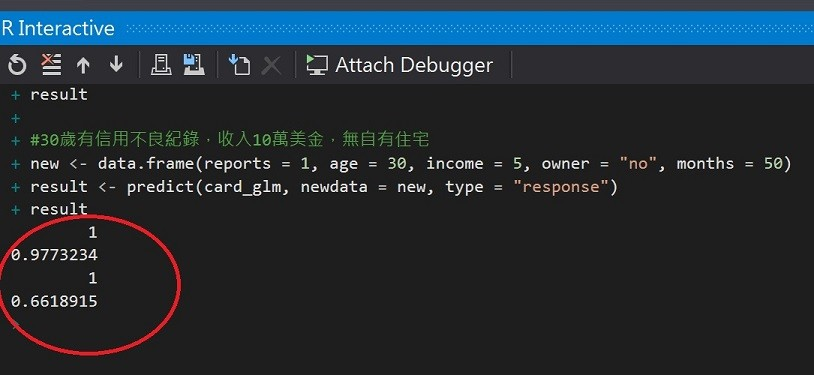## 混淆矩陣(confusion matrix)及準確度(Accuracy)

``````#(4)測試模型
#將資料分為訓練與測試組

#取得總筆數
n <- nrow(bankcard)
#設定隨機數種子
set.seed(1117)
#將數據順序重新排列
newbankcard <- bankcard[sample(n),]

#取出樣本數的idx
t_idx <- sample(seq_len(n), size = round(0.7 * n))

#訓練資料與測試資料比例: 70%建模，30%驗證
traindata <- newbankcard[t_idx,]
testdata <- newbankcard[ - t_idx,]
``````

``````# 重新建立羅吉斯迴歸模型
card_glm2 <- glm(formula = card ~ ., family = "binomial", data = traindata)
result <- predict(card_glm2, newdata = testdata, type = "response")

#(5)建立混淆矩陣(confusion matrix)觀察模型表現

#r假設我們認定核準率60%以上才視為核卡，其餘是為拒件或補件
result_Approved <- ifelse(result > 0.6, 1, 0)
cm <- table(testdata\$card, result_Approved, dnn = c("實際", "預測"))
cm
``````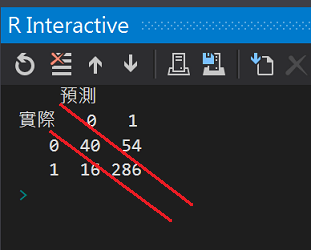``````#(6)準確率
#計算核準卡正確率
cm / sum(cm[, 2])

#計算拒補件正確率
cm / sum(cm[, 1])

#整體準確率(對角線元素總和/所有觀察值總和)
accuracy <- sum(diag(cm)) / sum(cm)
accuracy
``````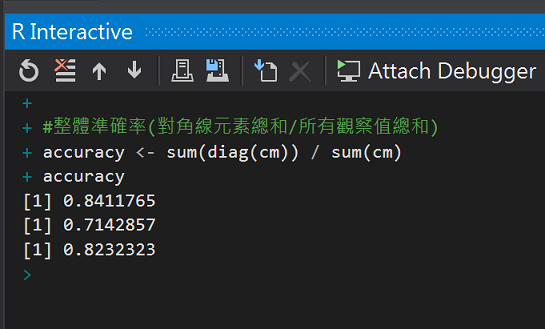• 核準卡準確率84%
• 拒補件準確率71%
• 整體準確率82%

• TPR = TP/(TP+FN) 實際核准的申請中，被模型說中核准的命中率 (hit rate)
• FPR = FP/(FP+TN) 實際拒捕件的申請中，被模型預測錯誤的比例，也稱假警報率 (false alarm rate)

• 1-specificity=FPR，沒有假警報的機率。
• Sensitivity=TPR(True positive rate),被模型說中核准的命中率。

``````#畫ROC曲線
library("ROCR")
pred <- prediction(result, testdata\$card)
perf <- performance(pred, measure = "tpr", x.measure = "fpr")
#計算AUC
auc <- performance(pred, "auc")

#畫圖
plot(perf, col = rainbow(7), main = "ROC curve", xlab = "Specificity(FPR)", ylab = "Sensitivity(TPR)")
#AUC = 0.5
abline(0, 1)
#實際AUC值
text(0.5, 0.5, as.character(auc@y.values[]))
``````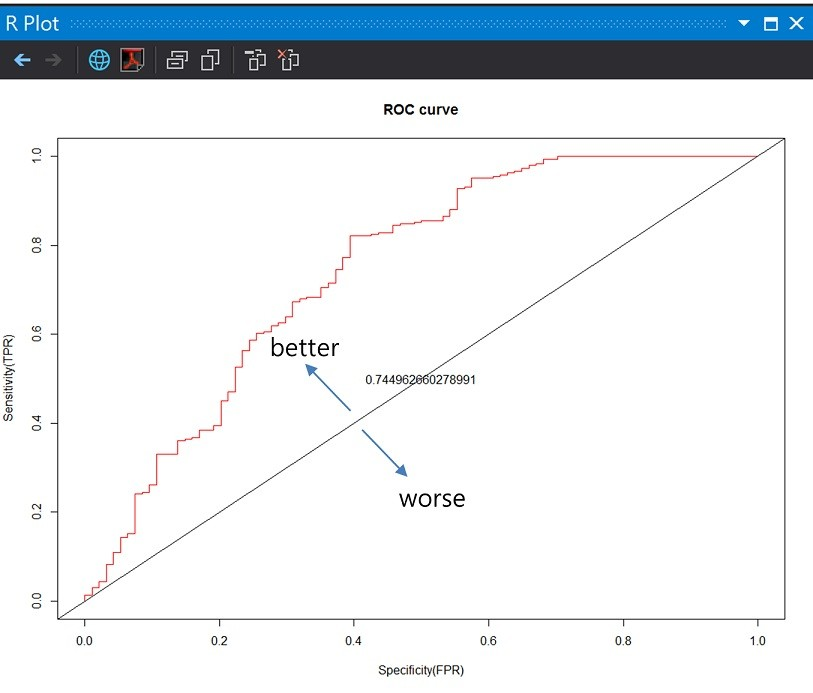ROC曲線下方的面積稱為AUC(Area under the Curve of ROC)

## 參考:

wiki Confusion matrix

wiki Logistic regression

AER Creditcard dataset

ROC曲線

http://archive.ics.uci.edu/ml/datasets/default+of+credit+card+clients2014.10攝於Ronda,spain

R語言與機器學習見面會30